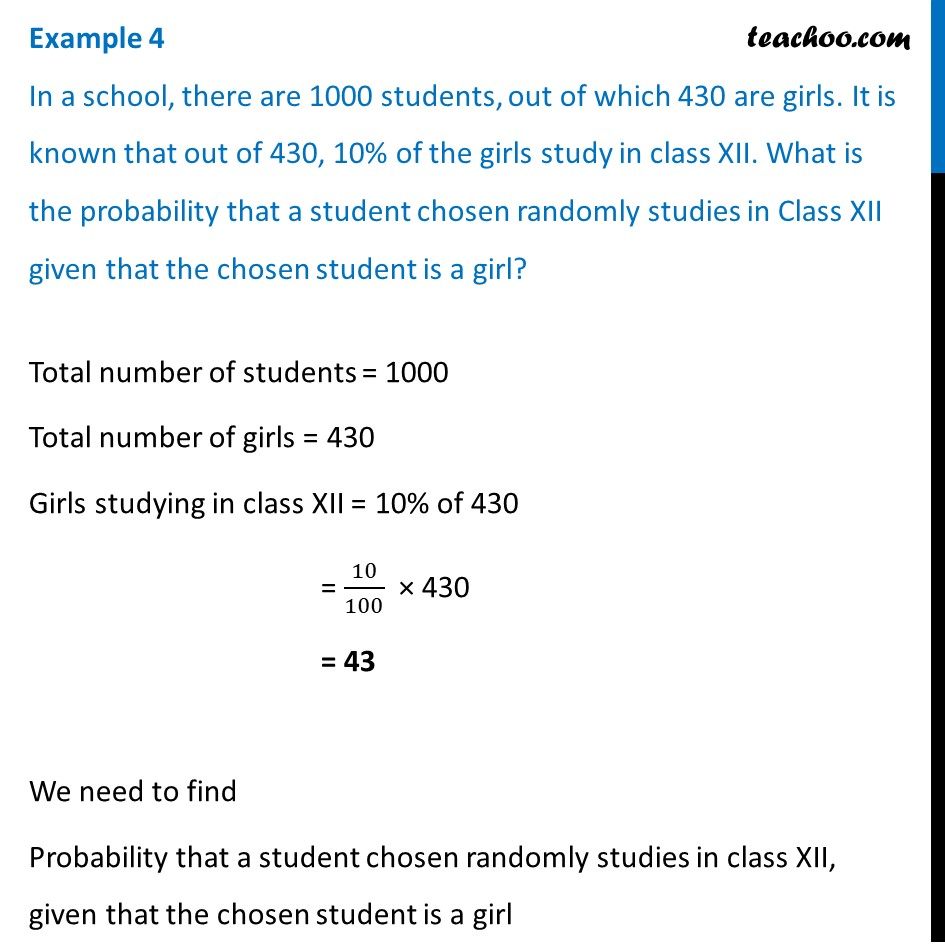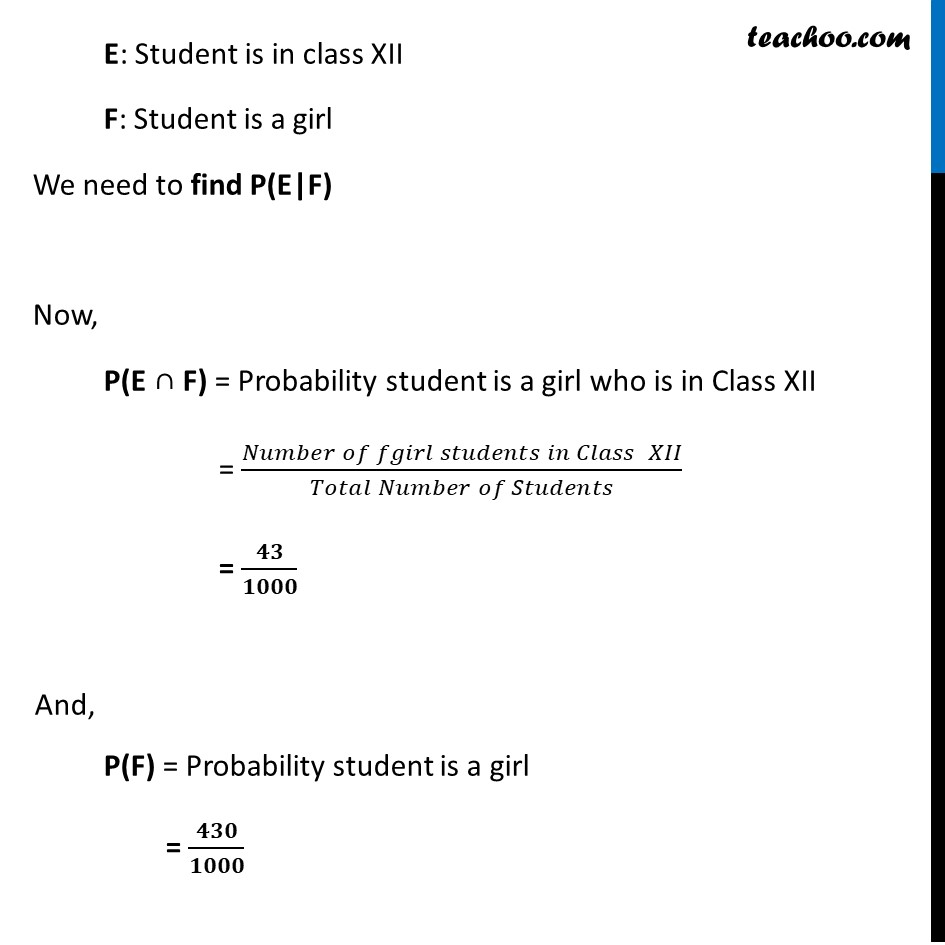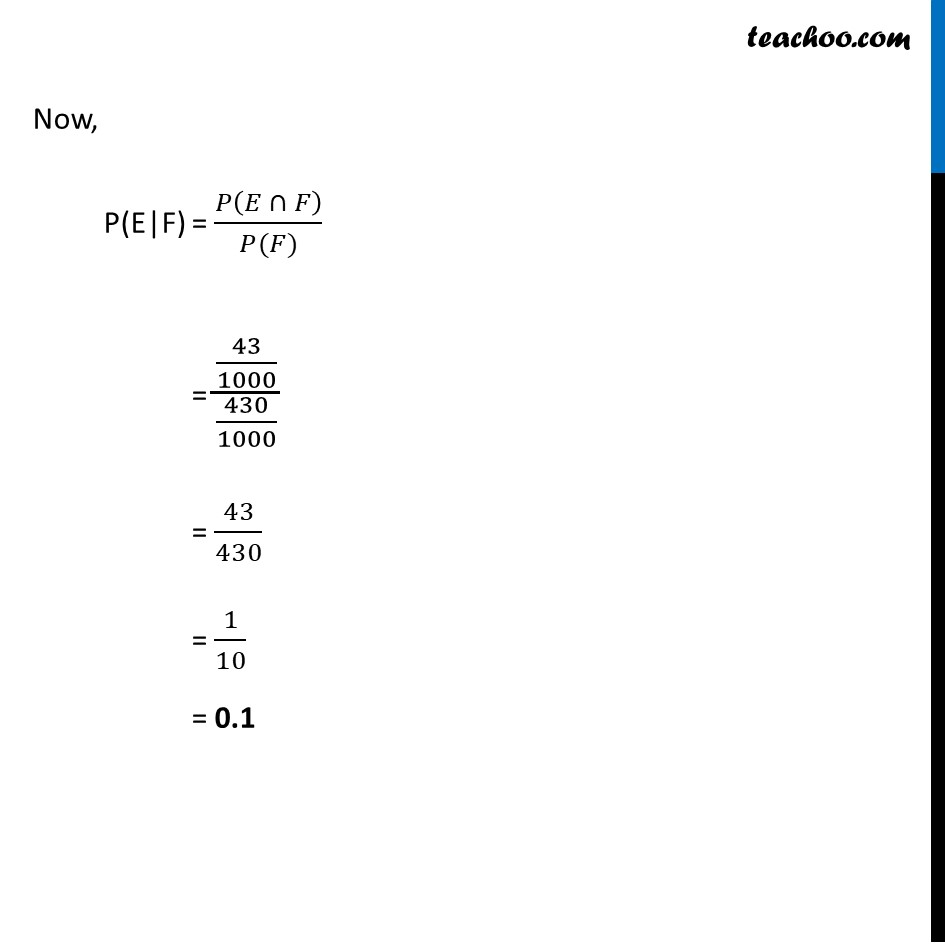Examples

Chapter 13 Class 12 Probability
Serial order wiseLearn in your speed, with individual attention - Teachoo Maths 1-on-1 Class

### Transcript

Example 4 In a school, there are 1000 students, out of which 430 are girls. It is known that out of 430, 10% of the girls study in class XII. What is the probability that a student chosen randomly studies in Class XII given that the chosen student is a girl? Total number of students = 1000 Total number of girls = 430 Girls studying in class XII = 10% of 430 = 10/100 × 430 = 43 We need to find Probability that a student chosen randomly studies in class XII, given that the chosen student is a girl E: Student is in class XII F: Student is a girl We need to find P(E|F) Now, P(E ∩ F) = Probability student is a girl who is in Class XII = (𝑁𝑢𝑚𝑏𝑒𝑟 𝑜𝑓 𝑓𝑔𝑖𝑟𝑙 𝑠𝑡𝑢𝑑𝑒𝑛𝑡𝑠 𝑖𝑛 𝐶𝑙𝑎𝑠𝑠 " " 𝑋𝐼𝐼)/(𝑇𝑜𝑡𝑎𝑙 𝑁𝑢𝑚𝑏𝑒𝑟 𝑜𝑓 𝑆𝑡𝑢𝑑𝑒𝑛𝑡𝑠) = 𝟒𝟑/𝟏𝟎𝟎𝟎 And, P(F) = Probability student is a girl = 𝟒𝟑𝟎/𝟏𝟎𝟎𝟎 Now, P(E|F) = 𝑃(𝐸 ∩ 𝐹)/(𝑃(𝐹)) = (43/1000)/(430/1000) = 43/430 = 1/10 = 0.1